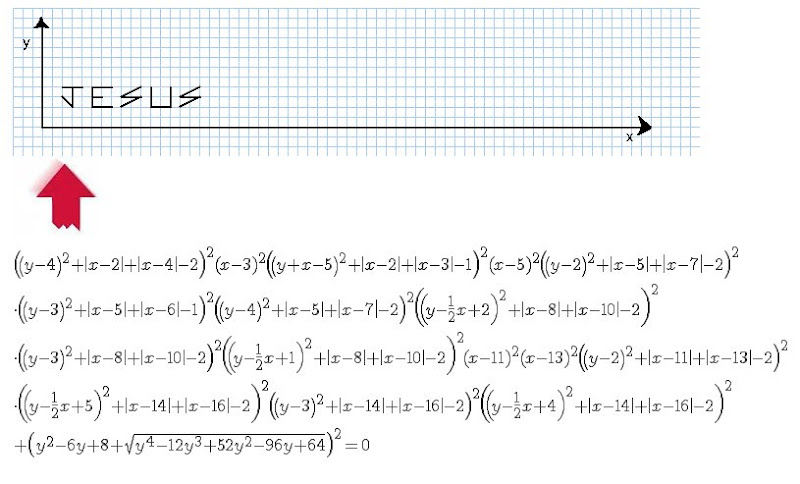# The equation of life

The inverse graphical calculator creates a graph out of words you input then gives you the equation to generate the graph.
Here is the equation for Jesus.